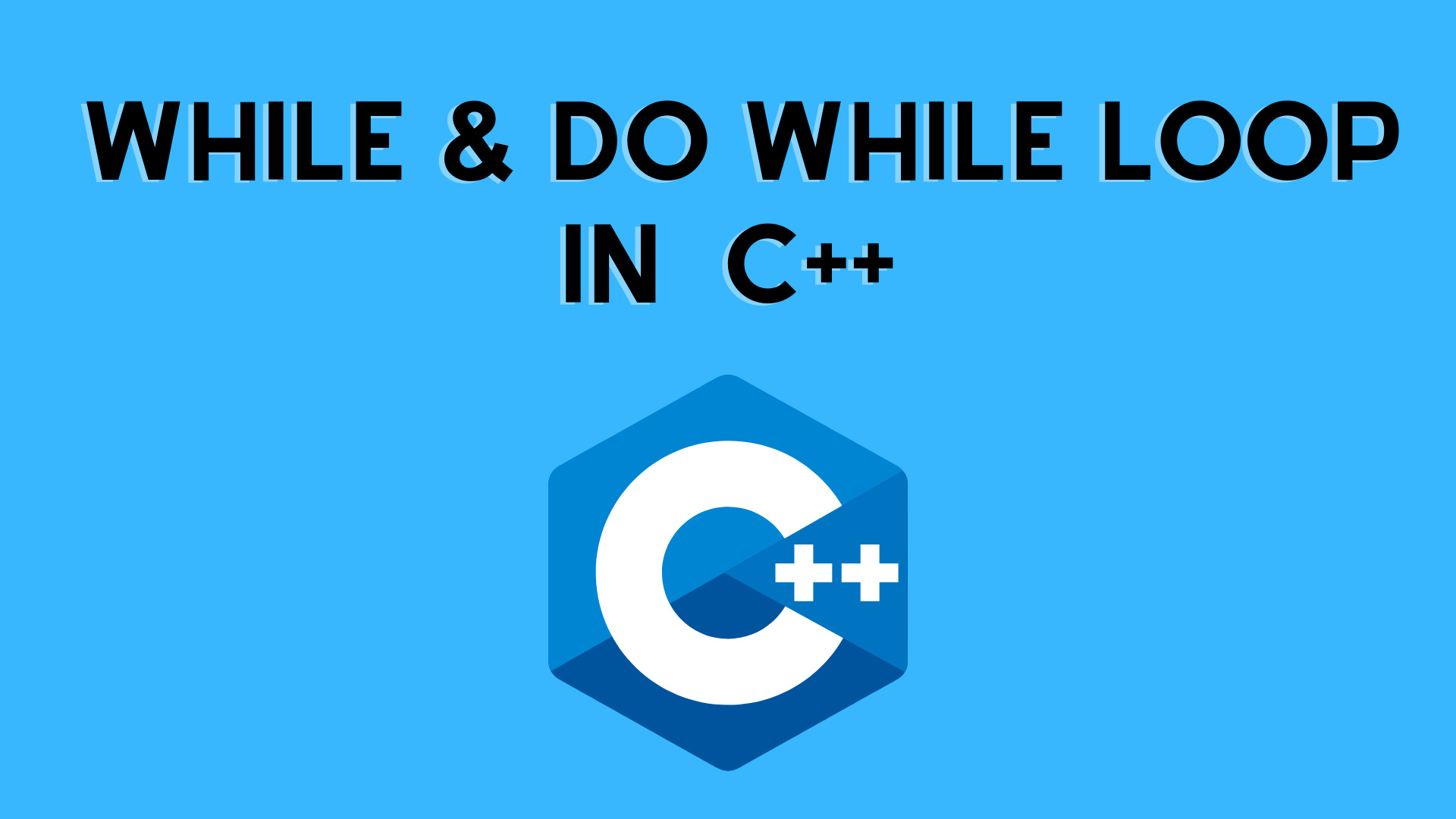## While and do…while loop with Example | C++ Programming

In this tutorial, we will learn the use of while and do…while loops in C++ programming with the help of some examples.

Loops are used in computer programming to repeat a section of code.

• Let’s imagine we wish to broadcast a message 100 times. We may then use a loop instead of writing the print statement 100 times.
• That was only a simple example; loops can help us attain far greater efficiency and sophistication in our applications.

There are 3 types of loops in C++.

1. for loop
2. while loop
3. do…while loop

We learned about the C++ for loop in the last tutorial. We’ll learn about while and do…while loops in this section.

While studying loops, we discovered that the number of iterations is known ahead of time, i.e. the number of times the loop body must be executed. While loops are employed in circumstances where the exact number of loop iterations is unknown ahead of time. On the basis of the test condition, the loop execution is terminated.

Syntax: As previously indicated, a loop consists mostly of three statements: initialization expression, test expression, and update expression. The placement of these three statements varies between the three loops – While, and do-while, For.

Syntax:

while (test-expression)
{
// statements
}

Working of while loop:

• Control is trapped in a while loop.
• The flow then jumps to Condition, which is tested.
• If the condition is true, the flow is directed to the Body.
• If the condition returns false, the flow exits the loop.
```// C++ program to illustrate while loop
#include <iostream>
using namespace std;

int main()
{
// initialization expression
int i = 1;

// test expression
while (i < 6)
{
cout << "Developers Dome\n";

// update expression
i++;
}

return 0;
}
```

Output:

Developers Dome
Developers Dome
Developers Dome
Developers Dome
Developers Dome

Points to Remember:

• When the number of iterations is known ahead of time, i.e. the number of times the loop body must be executed, use for loop.
• When the exact number of iterations is unknown but the loop termination condition is known, use while loops.
• If the code must be executed at least once, such as in menu-driven programs, use the do-while

Example: Display Numbers from 1 to 5

```#include <iostream>

using namespace std;

int main() {
int i = 1;

while (i <= 5) {
cout << i << " ";
++i;
}

return 0;
}```

Output

1 2 3 4 5

Example 2:

```#include <iostream>
using namespace std;

int main()
{
int i = 1;
while (i > -5) {
cout << i << "\n";
i--;
}

return 0;
}
```

Output:

1
0
-1
-2
-3
-4

Example: Infinitive While Loop.

```#include <iostream>

using namespace std;

int main() {
while (i <= 5) {
cout <<"Devlopers Dome";

}

return 0;
}```

Output:

## Do While Loop

The loop execution in do-while loops is also terminated based on the test condition. The key distinction between a do-while loop and a while loop is that the condition in a do-while loop is evaluated at the end of the loop body, whereas the other two loops are entry-controlled loops.
Note that, regardless of the test condition, the loop body will execute at least once in a do-while loop.

Syntax:

// initialization expression;
do
{
// statements
// update_expression;
} while (test_expression);

Example:-Display Numbers from 1 to 5 using while loop

```// C++ Program to print numbers from 1 to 5

#include <iostream>

using namespace std;

int main() {
int i = 1;

// while loop from 1 to 5
while (i <= 5) {
cout << i << " ";
++i;
}

return 0;
}```

Example:-Display Developers Dome using do-while loop

```// C++ program to illustrate do-while loop
#include <iostream>
using namespace std;

int main()
{
int i = 2; // Initialization expression

do
{
// loop body
cout << "Developers Dome\n";

// update expression
i++;

} while (i < 1); // test expression

return 0;
}
```

Output:

Developers Dome

## Getting out of a loop

When running a loop, it is sometimes required to skip a section of the loop or to exit the loop as soon as a given condition is satisfied, which is known as jumping out of the loop. Jumping from one statement to the next within a loop, as well as jumping out of the loop, is possible in C.

1) Break Statement
When a break statement is found within a loop, the loop is quit immediately and the program continues with the statement immediately following the loop.

2) Continue Statement
It directs the control to the test condition before continuing the loop operation. When the pointer comes across the word continue, it leaves the current loop cycle and begins the next one.

We, Will, discuss it further.

You may like:

C++ For loop With Example

Type Conversion In C++ Programming

Input and Output in C++ | C++ Programming

C++ Basic Syntax | C++ Tutorial

Features of C++ | C++ Tutorial

Operators In C++ Programming